# The circle as a challenge and an inspiration resource

The circle

Theme: practical application on the geometric shape of circle;
Key words: the circle,  the radius, the diameter, the circumference .

Content: form 7 grade core curriculum in Romania.

Objectives: At the end of this lesson students will be able to:

• recognize circle’s elements such as radius, diameter and circumference into a geometric configuration;
• to use circle’s  properties regarding  the circomference into  practical situations;
• to claculate distances and lenghts using the circumference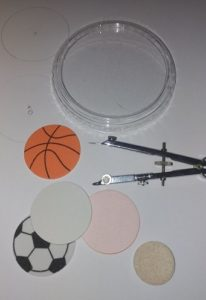Definition: As you may know a circle is a geometric shape formed by the points from a plan, situated to the same distance from a fix point named the centre of the circle.

Exercice 1) Please write the name of four objects that  include a surface of a circle and indicate your nickmane in the following form.

Geometric elements tied to the circle:

The radius(R) is a line segment between  the center of the circle and any point situated on the circle.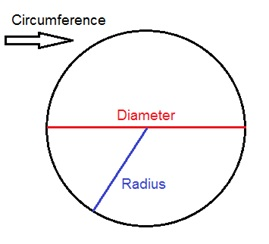The diameter(D) is a line segment with both extremities on the circle, that contains the center of the circle.

The circumference(L) or the lenght of the circle is the lenght of a string that surounds completly a circle.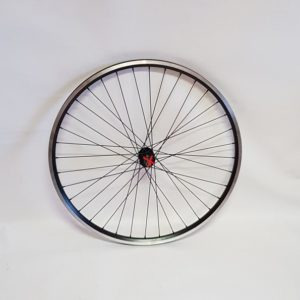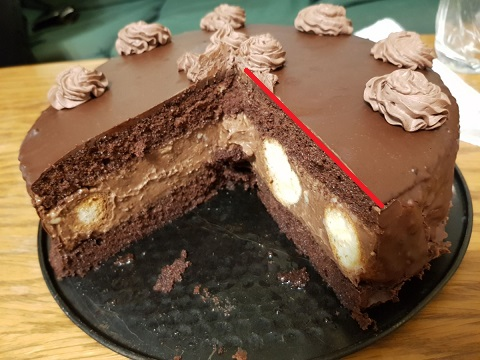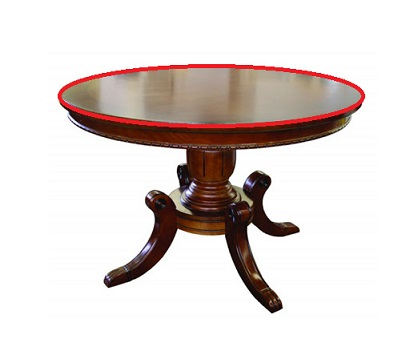Theoreme on circle’s circomference

During practice we observed that the ratio between the circumference and the diameter is the same value. In fact the value is the famous number pi:

π=L/D=3.141592….

According to that observation, the circumference of the circle is

L=2πR=πD

Practical issue:

So if I have a wheel with an diameter of 1,5 m, how many times will it rolls in my courtyard from the entrance gate to the garage, considering that the distance between the gate and the garage 10 m.

The calculus for the number of rolls:

D=1,5 m

This weel circumference is L=π⋅D=3,14⋅1,5=4,71 m

The number of spins is a ratio: n= distance/L=10/4,71=2,12

Answer: the wheel will roll completely 2 times.